## Quiz 10 : Special Pricing PracticesLooking for Economics Homework Help?# Quiz 10 : Special Pricing Practices

There are only two firms in the Widget industry. The total demand for widgets is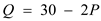. The two firms have identical cost functions,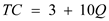. The two firms agree to collude and act as though the industry were a monopoly. The Price and Quantity at which this cartel would maximize its profit can be calculated by inverting the demand function for P: Given: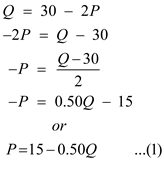Total Revenue for both firms can be calculated by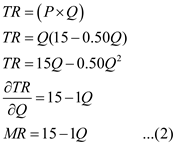Total Cost of both firms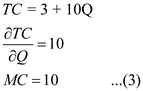For Maximum Profit MC = MR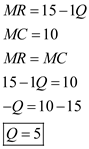From (1) we can find equilibrium P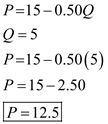Thus, we can say that Profit Maximizing price is P=12.5 and Profit Maximizing quantity is Q = 5.

The given statement state that "If a company sets its price on the basis of a cost-plus calculation, it cannot possibly suffer a loss on its products.", This statement is false because company profit depends on the market price. If the market price is lower than the company's price then company have to bear the losses. Thus, it is False , that setting prices on the basic of cost-plus calculation cannot leads to loss.

The demand of a good is the quantity necessary of that good by the persons of an economy. The prerequisite turn out to be a demand when individuals are ready and able to pay for the good. Supply of a good is the amount of a good that the manufacturers are ready to sell in a market. Equilibrium price is the price at which demand and supply are equal. Similarly, equilibrium quantity is the quantity of a good exchanged in the market after arbitration. a. The demand function and the cost function is given as: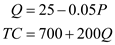1. The price and quantity if B well maximize profits is calculated as follows: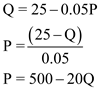First, calculate total revenue (TR) by multiplying price and quantity and calculate marginal revenue (MR) as follows: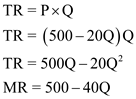Calculate marginal cost (MC) as follows: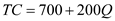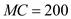Now, using the profit maximizing condition calculate Q as follows: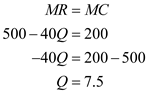Now substitute the values of Q in the given demand function and solve for P as follows: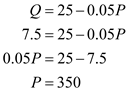Hence, if B well maximize profits then the price would be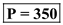and the quantity would be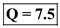. 2. In order to find price and quantity when revenue is maximized, first find the average revenue (AR) by dividing the TR by Q as follows: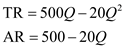Now, calculate average cost (AC) by dividing TC by Q as follows: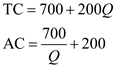Now, using the revenue maximizing condition, the price and quantity is calculated as follows: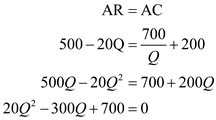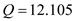Substitute the value of Q in the demand function and solve for P as follows: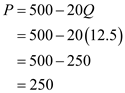Hence, the revenue maximizing price is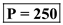and quantity is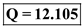. 3. In this case, minimum profit is required to be \$300, before maximizing revenue. So when P = 350 and Q = 7.5 (calculated in part 1.) the profit will be calculated as: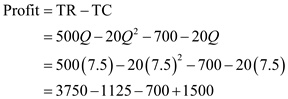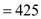Since, profit estimated here is \$425, thus satisfies the minimum profit condition. Now consider the revenue maximization (calculated in part 2.). Hence,andb. The demand function and the cost function is given as: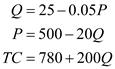1. The profit maximizing price and quantity is calculated as follows: Since, the demand function is same as given in part (a), therefore the TR and MR will be same. Now calculate MC as follows: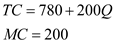It shows that MC is also same as calculated in part (a). Thus, the price and quantity will be same as calculated in part (a). Hence, the profit maximizing price isand quantity is. 2. In order to find price and quantity when revenue is maximized, first find the AR and AC. Since, TR is same as calculated in part (a), so AR will also be same. Now calculate average cost (AC) by dividing TC by Q as follows: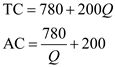Now using the revenue maximizing condition, the price and quantity is calculated as follows: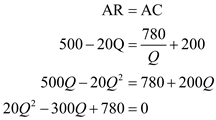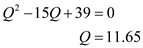Substitute the value of Q in the demand function and solve for P as follows: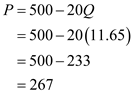Hence, the revenue maximizing price is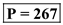and quantity is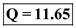. 3. In this case, minimum profit is required to be \$300, before maximizing revenue. So when P = 350 and Q = 7.5 (calculated in part a (1)) the profit will be calculated as: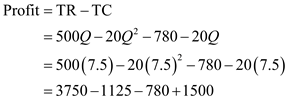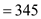Since, profit estimated here is \$345, thus satisfies the minimum profit condition. Now consider the revenue maximization (calculated in b (2)). Hence, the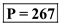and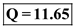c. The answer is same in a(1) and b(1) because the MR and MC are same and thus, the profit maximizing price and quantity are same. On the other hand, answer is not same in a(3) and b(3) because AC is different in both cases.